# CBSEtips.in

## Thursday, 25 February 2021

### CBSE Class 7 Maths - MCQ and Online Tests - Unit 5 - Lines and Angles

#### CBSE Class 7 Maths – MCQ and Online Tests – Unit 5 – Lines and Angles

Every year CBSE students attend Annual Assessment exams for 6,7,8,9,11th standards. These exams are very competitive to all the students. So our website provides online tests for all the 6,7,8,9,11th standards’ subjects. These tests are also very effective and useful for those who preparing for any competitive exams like Olympiad etc. It can boost their preparation level and confidence level by attempting these chapter wise online tests.

These online tests are based on latest CBSE syllabus. While attempting these, our students can identify their weak lessons and continuously practice those lessons for attaining high marks. It also helps to revise the NCERT textbooks thoroughly

#### CBSE Class 7 Maths – MCQ and Online Tests – Unit 5 – Lines and Angles

Question 1.
The measure of the complement of the angle 46° is
(a) 90°
(b) 44°
(c) 16°
(d) 136°

Hint:
Complementary angle = 90 – given angle
? Complement of 46 = 90 – 46 = 44

Question 2.
The sum of the measures of two supplementary angles is
(a) 90°
(b) 180°
(c) 360°
(d) none of these

Hint:
Definition of supplementary angles.

Question 3.
The sum of the measures of two complementary angles is
(a) 180°
(b) 60°
(c) 45°
(d) 90°

Hint:
Definition of complementary angles

Question 4.
The measure of the complement of the angle 30° is
(?) 30°
(b) 16°
(c) 60°
(d) 160°

Hint:
90° – 30° = 60°.

Question 5.
When the sum of the measures of two angles is 90°, the angles are called
(?) supplementary angles
(b) complementary angles
(d) vertically opposite angles

Answer: (b) complementary angles
Hint:
Definition of complementary angles

Question 6.
Which of the following statements is true?
(a) Two acute angles can be complementary to each other
(b) Two obtuse angles can be complementary to each other
(c) Two right angles can be complementary to each other
(d) One obtuse angle and one acute angle can be complementary to each other

Answer: (a) Two acute angles can be complementary to each other

Question 7.
What is the measure of the complement of the angle 80°?
(a) 10°
(b) 100°
(c) 36°
(d) 20°

Hint:
90° – 80° = 10°.

Question 8.
Which pair of the following angles are complementary?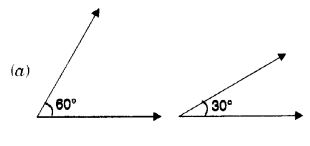Hint:
60°+ 30° = 90°.

Question 9.
Which of the following pairs of angles is not a pair of complementary angles?
(a) 60°, 30°
(b) 66°, 34°
(c) 0°, 90°
(d) 160°, 30°

Answer: (d) 160°, 30°
Hint:
150° + 30° = 180° ? 90°.

Question 10.
What is the measure of the complement of the angle 90°?
(?) 90°
(b) 0°
(c) 180°
(d) 46°

Hint:
90° – 90° = 0°.

Question 11.
When the sum of the measures of two angles is 180°, the angles are called
(b) complementary angles
(c) vertically opposite angles
(d) supplementary angles

Answer: (d) supplementary angles
Hint:
Definition of supplementary angles.

Question 12.
The measure of the angle which is equal to its complement is
(a) 30°
(b) 60°
(c) 46°
(d) 90°

Hint:
x° + x° = 90° ? x° = 45°.

Question 13.
The measure of the supplement of the angle 120° is
(a) 30°
(b)45°
(c) 60°
(d) 90°

Hint:
180° – 120° = 60°.

Question 14.
Which of the following statements is true?
(a) Two acute angles can be supplementary.
(b) Two right angles can be supplementary.
(c) Two obtuse angles can be supplementary.
(d) One obtuse angle and one acute angle cannot be supplementary

Answer: (b) Two right angles can be supplementary.

Question 15.
Which of the following pairs of angles is not a pair of supplementary angles?
(a) 90°, 90°
(b) 32°, 58°
(c) 0°, 180°
(d) 76°, 104°

Answer: (b) 32°, 58°
Hint:
32° + 58° = 90° ? 180°.

Question 16.
What is the measure of the supplement of the angle 0°?
(a) 45°
(b) 90°
(c) 120°
(d) 180°

Hint:
180° – 0° = 180°.

Question 17.
Which pair of the following angles are not supplementary?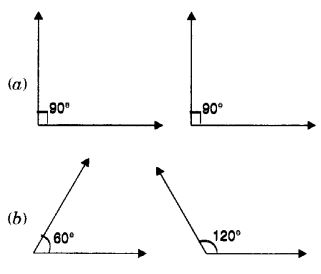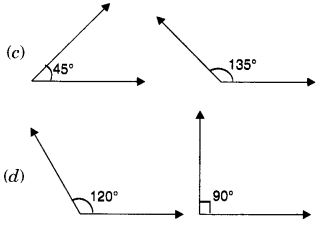Hint:
120° + 90° = 210° ? 180°.

Question 18.
The measure of the supplement of the angle 90° is
(a) 45°
(b) 60°
(c) 30°
(d) 90°

Hint:
180° – 90° = 90°.

Question 19.
The measure of the angle which is equal to its supplement is
(a) 30°
(b) 45°
(c) 90°
(d) 60°

Hint:
x° + x° = 180° ? x° = 90°.

Question 20.
The measure of the supplement of the angle 179° is
(a) 1°
(b) 2°
(c) 3°
(d) 4°

Hint:
180° – 179° – 1°.

Question 21.
Which of the following statements is true?
(a) Two adjacent angles can be complementary.
(b) Two adjacent angles cannot be supplementary
(c) An acute angle cannot be adjacent to an obtuse angles.
(d) Two right angles cannot be adjacent angles

Answer: (a) Two adjacent angles can be complementary.

Question 22.
The angles in a linear pair are
(a) complementary
(b) supplementary
(c) not adjacent angles
(d) vertically opposite angles

Hint:
Definition of a linear pair of angles.

Question 23.
Which of the following statements is true?
(a) Two acute angles can form a linear pair.
(b) Two obtuse angles can form a linear pair
(c) Two right angles can form a linear pair
(d) One obtuse angle and one acute angle cannot form a linear pair

Answer: (c) Two right angles can form a linear pair

Question 24.
Which of the following pairs of angles form a linear pair?Hint:
120° + 60° = 180°.

Question 25.
Which of the following statements is false?
(a) When a transversal cuts two parallel lines, each pair of corresponding angles are equal.
(b) When a transversal cuts two parallel lines, each pair of alternate interior angles are equal.
(c) When a transversal cuts two parallel lines, each pair of interior angles on the same side of the transversal are supplementary.
(d) A transversal cuts two parallel lines in three points

Answer: (d) A transversal cuts two parallel lines in three points

Question 26.
In the following figure, ? AOB and ? BOC are(a) complementary angles
(b) supplementary angles
(d) none of these

Answer: (d) none of these

Question 27.
In the following figure, reflex ? AOB is equal to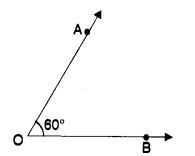(a) 60°
(b) 120°
(c) 300°
(d) 360°

Hint:
?AOB = 360° – 60° = 300°.

Question 28.
The sum of the measures of the angles in a linear pair is
(a) 90°
(b) 180°
(c) 360°
(d) none of these

Hint:
Definition of a linear pair of angles.

Question 29.
Which of the following statements is false?
(a) Two vertically opposite angles can be acute
(b) Two vertically opposite angles can be obtuse
(c) Two vertically opposite angles can be right angles
(d) Two vertically opposite angles may be unequal

Answer: (d) Two vertically opposite angles may be unequal

Question 30.
Which of the following statements is false?
(a) When a transversal cuts two lines, such that pairs of corresponding angles are equal, then the lines have to be parallel.
(b) When a transversal cuts two lines such that pairs of alternate interior angles are equal, then the lines have to be parallel.
(c) When a transversal cuts two lines such that pairs of interior angles on the same side of the transversal are supplementary, then the lines have to be parallel.
(d) When a transversal cuts two lines such that pairs of interior angles on the same side of the transversal are complementary, then the lines have to be parallel

Answer: (d) When a transversal cuts two lines such that pairs of interior angles on the same side of the transversal are complementary, then the lines have to be parallel

Question 31.
See the figure of Q. 32 above. Which pair of angles are alternate interior angles?
(a) ?1, ?5
(b) ?2, ?6
(c) ?3, ?7
(d) ?3, ?5

Answer: (d) ?3, ?5
Hint:
Definition of alternate interior angles.

Question 32.
In the following figure, a transversal cuts two parallel lines l and m at points G and H respectively and the angles thus formed are marked. If ?1 is an acute angle, then, which of the following statements is false?(?) ?1 + ?2 = 180°
(b) ?2 + ?5 = 180°
(c) ?3 + ?8 = 180°
(d) ?2 + ?6 = 180°.

Answer: (d) ?2 + ?6 = 180°
Hint:
?2 = ?6, corresponding angles.

Question 33.
In the following figure, a transversal c intersects two parallel lines a and b at A and B respectively and the angles formed at A and B are marked. Tell which of the following pairs of angles need not be equal?(a) ?1, ?2
(b) ?1, ?3
(c) ?1, ?5
(d) ?2, ?8

Answer: (a) ?1, ?2
Hint:
?1, ?2 simply make a linear pair.

Question 34.
Which of the following pairs of angles are not adjacent angles?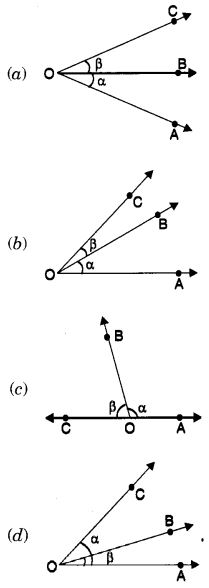Question 35.
Find the measure of the angle which is double of its complementary angle?
(a) 60°
(b) 30°
(c) 45°
(d) 120°

Hint:
x° = 2(90° – x°) ? x° = 60°.

Question 36.
Find the measure of the angle which is half of its supplementary angle?
(a) 60°
(b) 120°
(c) 90°
(d) 45°

Hint:
x° = $$\frac { 1 }{ 2 }$$(180° – x°) ? x° = 60°.

Question 37.
In the following figure, if ?1 + ?3 = 120°, then ?2 + ?4 is equal to(a) 60°
(b) 120°
(c) 240°
(d) 80°

Hint:
?2 + ?4 = 360°- (?1 + ?3) = 360°- 120° = 240°

Question 38.
In the following figure, if ?1 + ?2 = 100°, then the measure of ?4 is equal to(a) 50°
(b) 100°
(c) 80°
(d) 130°

Hint:
?1 = ?2; ?1 + ?2 = 100°
? ?1 = 50°
? ?4 = 180° – 50° = 130°.

Question 39.
In the following figure, ?1 + ?2 + ?3 = 230°, then the measure of ?4 is equal to(a) 130°
(b) 80°
(c) 65°
(d) 90°

Hint:
? 4 = 360° – 230° = 130°.

Question 40.
In the following figure EF || GH. Then, the measure of ?x is equal to(a) 30°
(b) 45°
(c) 60°
(d) 40°

Hint:
(x + 30°) + 120° = 180° ? x = 30°.

Question 41.
In the following figure, l || m. Find the measure of ? x.(a) 60°
(b) 45°
(c) 30°
(d) none of these

Hint:
x + x + 60° = 180° ? x = 60°.

Question 42.
In the following figure, AB || DG and BC || EF. Also, ?ABC = 60°. Then, the measure of ?DEF is(a) 30°
(b) 60°
(c) 45°
(d) 120°

Hint:
?DEF = ?DGC = ?ABC = 60°.

Question 43.
Find the value of x in the following figure, if l || m.(a) 30°
(b) 45°
(c) 60°
(d) none of these

Hint:
x + 120° = 180° ? x = 60°.

Question 44.
In the following figure, two straight lines AB and CD are intersecting each other at the point 0 and the angles thus formed at 0 are marked, then the value of ?x – ?y is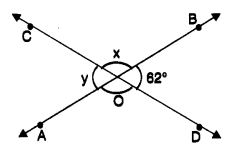(a) 56°
(b) 118°
(c) 62°
(d) 180°

Hint:
?x – ?y = (180° – 62°) – 62° = 118° – 62° = 56°.

Question 45.
In the following figure, tell which pair of angles are not corresponding angles?(a) ?1, ?5
(b) ?2, ?6
(c) ?3, ?7
(d) ?3, ?5

Answer: (d) ?3, ?5
Hint:
?3, ?5 are alternate interior angles.

Share: